International
Tables for
Crystallography
Volume A
Space-group symmetry
Edited by Th. Hahn

International Tables for Crystallography (2006). Vol. A, ch. 2.2, pp. 35-36

## Section 2.2.15.1. Maximal non-isomorphic subgroups6

Th. Hahna* and A. Looijenga-Vosb

aInstitut für Kristallographie, Rheinisch-Westfälische Technische Hochschule, Aachen, Germany, and bLaboratorium voor Chemische Fysica, Rijksuniversiteit Groningen, The Netherlands
Correspondence e-mail:  hahn@xtl.rwth-aachen.de

#### 2.2.15.1. Maximal non-isomorphic subgroups6

| top | pdf |

The maximal non-isomorphic subgroupsof a space groupare divided into two types: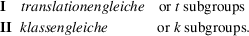For practical reasons, type II is subdivided again into two blocks:

IIa the conventional cells ofandare the same

IIb the conventional cell ofis larger than that of. 7

Block IIa has no entries for space groupswith a primitive cell. For space groupswith a centred cell, it contains those maximal subgroupsthat have lost some or all centring translations ofbut none of the integral translations (decentring' of a centred cell).

Within each block, the subgroups are listed in order of increasing index [i] and in order of decreasing space-group number for each value of i.

• (i) Blocks I and IIa

In blocks I and IIa, every maximal subgroupof a space groupis listed with the following information:

[i] HMS1 (HMS2, No.) Sequence of numbers.

The symbols have the following meaning:

[i]: index ofin(cf. the footnoteto Section 8.1.6);

HMS1: Hermann–Mauguin symbol of, referred to the coordinate system and setting of; this symbol may be unconventional;

(HMS2, No.): conventional short Hermann–Mauguin symbol of, given only if HMS1 is not in conventional short form, and the space-group number of.

Sequence of numbers: coordinate triplets ofretained in. The numbers refer to the numbering scheme of the coordinate triplets of the general position of(cf. Section 2.2.9). The following abbreviations are used:

 Block I (all translations retained): Number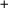Coordinate triplet given by Number, plus those obtained by adding all centring translations of. (Numbers)The same, but applied to all Numbers between parentheses. Block IIa (not all translations retained):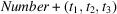Coordinate triplet obtained by adding the translation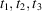to the triplet given by Number.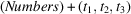The same, but applied to all Numbers between parentheses.

In blocks I and IIa, sets of conjugate subgroups are linked by left-hand braces. For an example, see space group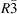(148) below.

#### Examples

 (1)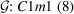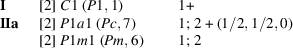where the numbers have the following meaning: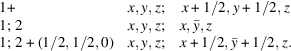(2)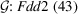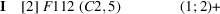where the numbers have the following meaning: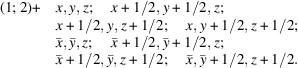(3)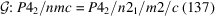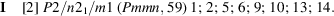Operations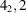and c, occurring in the Hermann–Mauguin symbol of, are lacking in. In the unconventional tetragonal version'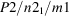of the symbol of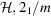stands for two sets of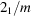(along the two orthogonal secondary symmetry directions), implying thatis orthorhombic. In the conventional orthorhombic version', the full symbol ofreads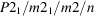and the short symbol Pmmn.

• (ii) Block IIb

Whereas in blocks I and IIa every maximal subgroupofis listed, this is no longer the case for the entries of block IIb. The information given in this block is:

[i] HMS1 (Vectors) (HMS2, No.)

The symbols have the following meaning:

[i]: index ofin;

HMS1: Hermann–Mauguin symbol of, referred to the coordinate system and setting of; this symbol may be unconventional;8

(Vectors): basis vectors a′, b′, c′ ofin terms of the basis vectors a, b, c of. No relations are given for unchanged axes, e.g.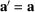is not stated;

(HMS2, No.): conventional short Hermann–Mauguin symbol, given only if HMS1 is not in conventional short form, and the space-group number of.

In addition to the general rule of increasing index [i] and decreasing space-group number (No.), the sequence of the IIb subgroups also depends on the type of cell enlargement. Subgroups with the same index and the same kind of cell enlargement are listed together in decreasing order of space-group number (see example 1 below).

In contradistinction to blocks I and IIa, for block IIb the coordinate triplets retained inare not given. This means that the entry is the same for all subgroupsthat have the same Hermann–Mauguin symbol and the same basis-vector relations to, but contain different sets of coordinate triplets. Thus, in block IIb, one entry may correspond to more than one subgroup,9 as illustrated by the following examples.

#### Examples

• (1)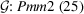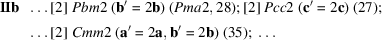Each of the subgroups is referred to its own distinct basis a′, b′, c′, which is different in each case. Apart from the translations of the enlarged cell, the generators of the subgroups, referred to a′, b′, c′, are as follows: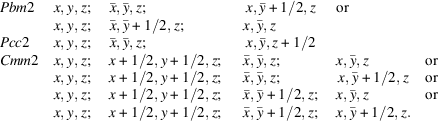There are thus 2, 1 or 4 actual subgroups that obey the same basis-vector relations. The difference between the several subgroups represented by one entry is due to the different sets of symmetry operations ofthat are retained in. This can also be expressed as different conventional origins ofwith respect to.

• (2)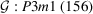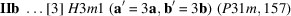The nine subgroups of type P31m may be described in two ways:

 (i) By partial decentring' of ninetuple cells (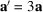,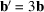,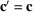) with the same orientations as the cell of the group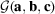in such a way that the centring points 0, 0, 0;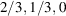;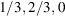(referred to a′, b′, c′) are retained. The conventional space-group symbol P31m of these nine subgroups is referred to the same basis vectors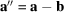,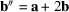,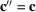, but to different origins; cf. Section 2.2.15.5. This kind of description is used in the space-group tables of this volume. (ii) Alternatively, one can describe the groupwith an unconventional H-centred cell (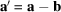,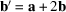,) referred to which the space-group symbol is H31m. Decentring' of this cell results in the conventional space-group symbol P31m for the subgroups, referred to the basis vectors a′, b′, c′. This description is used in Section 4.3.5.

• (iii) Subdivision of k subgroups into blocks IIa and IIb

The subdivision of k subgroups into blocks IIa and IIb has no group-theoretical background and depends on the coordinate system chosen. The conventional coordinate system of the space group(cf. Section 2.1.3) is taken as the basis for the subdivision. This results in a uniquely defined subdivision, except for the seven rhombohedral space groups for which in the space-group tables both rhombohedral axes' (primitive cell) and `hexagonal axes' (triple cell) are given (cf. Section 2.2.2). Thus, some k subgroups of a rhombohedral space group are found under IIa (klassengleich, centring translations lost) in the hexagonal description, and under IIb (klassengleich, conventional cell enlarged) in the rhombohedral description.

#### Example: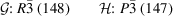Hexagonal axes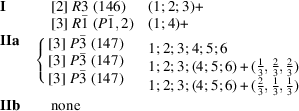Rhombohedral axes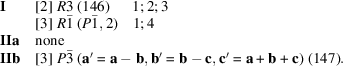Apart from the change from IIa to IIb, the above example demonstrates again the restricted character of the IIb listing, discussed above. The three conjugate subgroups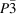of index  are listed under IIb by one entry only, because for all three subgroups the basis-vector relations betweenandare the same. Note the brace for the IIa subgroups, which unites conjugate subgroups into classes.